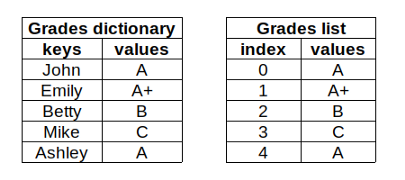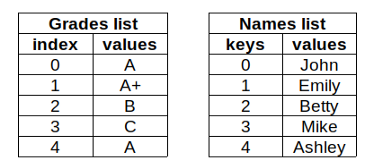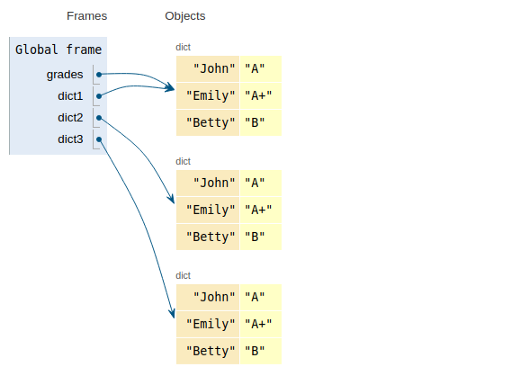# 掌握Python字典的12个例子

2020/11/15 19:09#### 1.创建字典

empty_dict = {}

grades = {'John':'A', 'Emily':'A+', 'Betty':'B', 'Mike':'C', 'Ashley':'A'}

{'Ashley': 'A', 'Betty': 'B', 'Emily': 'A+', 'John': 'A', 'Mike': 'C'}


#### 2.访问值

grades['John']
'A'

'B'


#### 3.访问所有值或所有键

keys方法用于获取所有键。

grades.keys()

dict_keys(['John', 'Emily', 'Betty', 'Mike', 'Ashley'])


grades.values()

dict_values(['A', 'A+', 'B', 'C', 'A'])


list(grades.values())
'A'


items方法返回键值对。

grades.items()

dict_items([('John', 'A'), ('Emily', 'A+'), ('Betty', 'B'), ('Mike', 'C'), ('Ashley', 'A')])


#### 4.更新或添加项

grades['Edward'] = 'B+'

{'Ashley': 'A',
'Betty': 'B',
'Edward': 'B+',
'Emily': 'A+',
'John': 'B',
'Mike': 'C'}


#### 5.使用新字典更新

grades = {'John':'A', 'Emily':'A+', 'Betty':'B', 'Mike':'C'}


grades.update(grades_new)

{'Betty': 'A', 'Emily': 'A+', 'John': 'B', 'Mike': 'C', 'Sam': 'A'}


#### 6.删除项

del(grades['Edward'])

'A'

'Betty': 'B', 'Emily': 'A+', 'John': 'B', 'Mike': 'C'}


#### 7.字典作为iterable

for i in grades:
print(i)

John
Emily
Betty
Mike


#### 8.字典生成式

{x: x**2 for x in range(5)}
{0: 0, 1: 1, 2: 4, 3: 9, 4: 16}

{word: len(word) for word in ['data','science','is','awesome']}
{'awesome': 7, 'data': 4, 'is': 2, 'science': 7}


iterable中的元素成为字典的键。这些值是根据字典生成式中的赋值确定的。

#### 9.从列表创建字典

a = [['A',4], ['B',5], ['C',11]]

dict(a)
{'A': 4, 'B': 5, 'C': 11}

b = [('A',4), ('B',5), ('C',11)]

dict(b)
{'A': 4, 'B': 5, 'C': 11}


#### 10.从字典到数据帧

Pandas的dataframe函数可用于使用字典创建数据帧。键变成列名，值变成行。

import numpy as np
import pandas as pd

dict_a = {'names':['Amber','John','Edward','Emily'],
'points':np.random.randint(100, size=4)}

df = pd.DataFrame(dict_a)
df#### 11.len和clear

len函数返回字典中的项数（即长度）。clear方法用于删除字典中的所有项，因此我们将得到一个空字典。

len(grades)
4

0


#### 12.复制字典

grades = {'John':'A', 'Emily':'A+', 'Betty':'B'}##### 好处：使用python3.9合并和更新操作符

Python3.9为字典提供了merge（“|”）和update（“|=”）运算符。我还没有安装Python 3.9，所以我将使用Python文档中的示例：

>>> x = {"key1": "value1 from x", "key2": "value2 from x"}
>>> y = {"key2": "value2 from y", "key3": "value3 from y"}
>>> x | y
{'key1': 'value1 from x', 'key2': 'value2 from y', 'key3': 'value3 from y'}
>>> y | x
{'key2': 'value2 from x', 'key3': 'value3 from y', 'key1': 'value1 from x'}


sklearn机器学习中文官方文档： http://sklearn123.com/0 评论
0 收藏
0